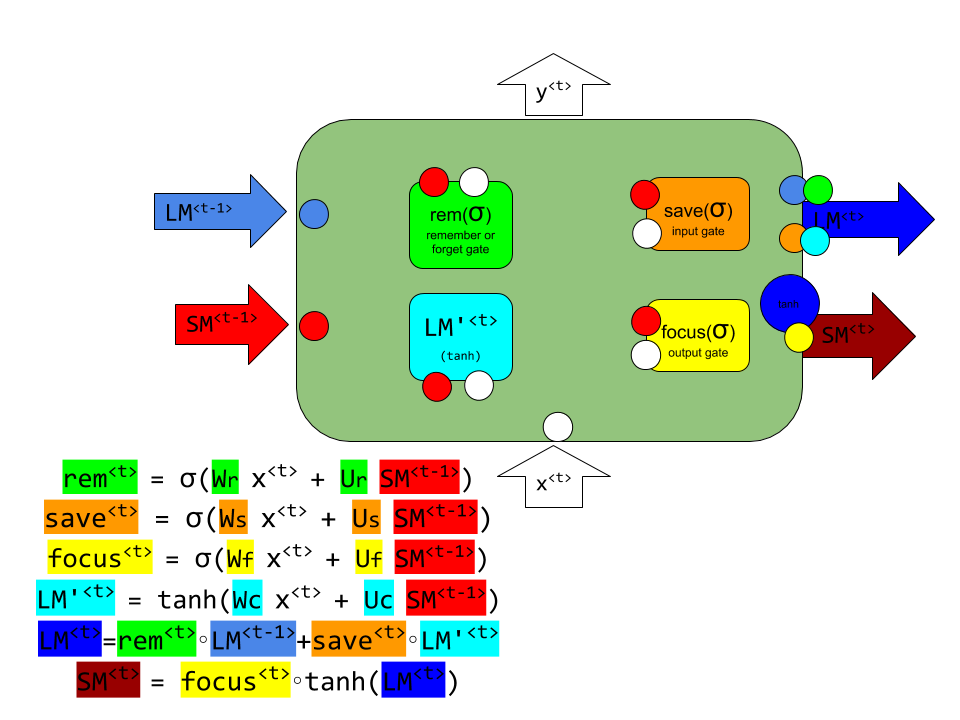We are defining a sequence of 20 numbers: 0 20 40 60 80 100 120 140 160 180 200 220 240 260 280 300 320 340 360 380 and memorize using Keras LSTM.

We would like to understand the final number of parameters for our model even though the model.summary() doesn’t explain much.

In the following code we feed the LSTM network directly with the values >20, so we are using the “relu” activation function.

Also, note the number of LSTM cells will be 20. We will have no batches, so n_batch = 1.

Our model is sequential. We reshaped the input data to have 20 time sequences, and 1 input feature.

import numpy as np
from keras.models import Sequential
from keras.layers import Dense
from keras.layers import LSTM

np.random.seed(0)

SLENG = 20 # sequence length
# numpy array
seq = np.arange(0, SLENG*SLENG, SLENG)
print(seq)
# 0  20  40  60  80 100 120 140 160 180 200 220 240 260 280 300 320 340 360 380

# model needs X as input and y as ouptut shapes
X = seq.reshape(1, SLENG, 1)
y = seq.reshape(1, SLENG)

# define LSTM configuration
n_neurons = SLENG
n_batch = 1
n_epoch = 1500

# create LSTM net
model = Sequential()
model.add(LSTM(n_neurons, activation="relu", input_shape=(SLENG, 1)))

print(model.summary())
# train LSTM
model.fit(X, y, epochs=n_epoch, batch_size=n_batch, verbose=0)
# evaluate
result = model.predict(X, batch_size=n_batch, verbose=0)
for value in result[0,:]:
print(value)



What do do next?

av = model.layers.get_weights()
W = model.layers.get_weights()
U = model.layers.get_weights()
b = model.layers.get_weights()

print("W", W.size)
print("U", U.size)
print("b", b.size)

units=SLENG
W_i = W[:, :units]
W_f = W[:, units: units * 2]
W_c = W[:, units * 2: units * 3]
W_o = W[:, units * 3:]

U_i = U[:, :units]
U_f = U[:, units: units * 2]
U_c = U[:, units * 2: units * 3]
U_o = U[:, units * 3:]

b_i = b[:units]
b_f = b[units: units * 2]
b_c = b[units * 2: units * 3]
b_o = b[units * 3:]


We have asked the model to show the layer info. In particular the W, U and b tensors. The output will be like this:

W 80
U 1600
b 80


This corresponds to the model.summary() method. If we calculate the total number of parameters for the LSTM we get 80 + 1600 + 80 = 1760.

_________________________________________________________________
Layer (type)                 Output Shape              Param #
=================================================================
lstm_42 (LSTM)               (None, 20)                1760
_________________________________________________________________
dense_42 (Dense)             (None, 20)                420
=================================================================
Total params: 2,180
Trainable params: 2,180
Non-trainable params: 0
_________________________________________________________________


But why there is 80 parameters for the W tensor and 1600 params for the U tensor and 80 parameters for the b tensor?

If you recall the image:There are three gates in LSTM cell and one unit for setting the new cell value (Long Memory). We marked it with LM’. There will be 4 * 20 = 80 parameters W in our LSMT layer, where 20 is the number of LSTM cells in our model.

Similarly there will be 80 b parameters in LSTM layer.

The number of U parameters is different. While W is same for all LSMT cells (W is connected with the input X, U is separate for each cell).

The number of U parameters would be 4* 20* 20 = 1600, because each LSTM cell has unique 4 * 20 parameters based on input shape.